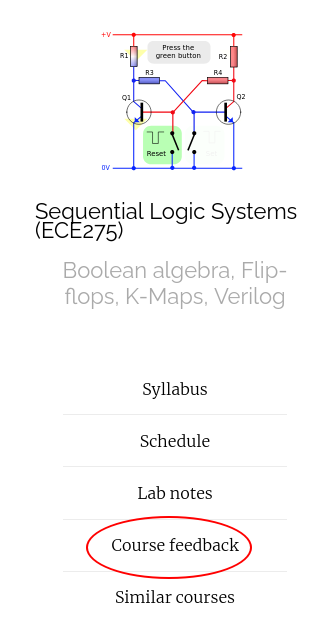## Announcemnts

• Midterm 2 postponed to Nov 15th instead of Nov 8th.
• HW 8 is posted is due next Wednesday (Nov 10th) at 9 AM before class.

## Ex2: Parity Checker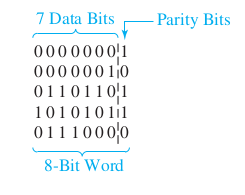## Ex2: Parity Checker using T flip-flops## Ex2: Parity Checker using J-K flip-flops## Ex2: Parity Checker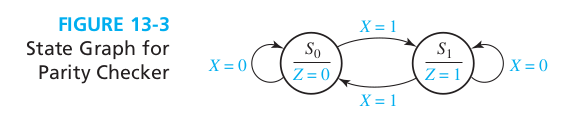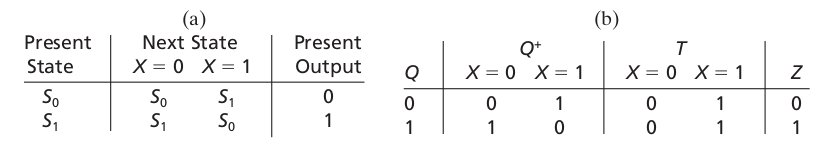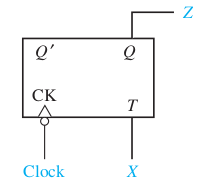## State reduction: Ex1

A sequential circuit has one input (X) and one output (Z). The circuit examines groups of four consecutive inputs and produces an output $$Z = 1$$ if the input sequence 0101 or 1001 occurs. The circuit resets after every four inputs. Find the Mealy state graph.

## State reduction: Ex1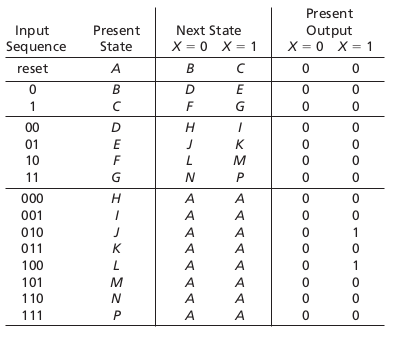## State reduction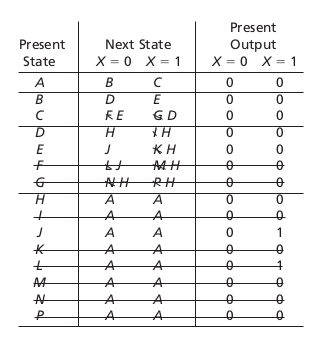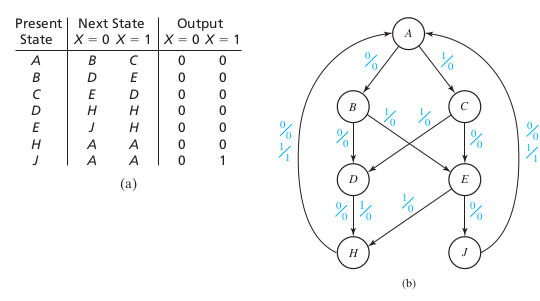## Equivalent states

Let $$N_1$$ and $$N_2$$ be sequential circuits (not necessarily different). Let $$\underline{X}$$ represent a sequence of inputs of arbitrary length. Then state p in $$N_1$$ is equivalent to state q in $$N_2$$ iff $$\lambda_1(p, \underline{X} ) = \lambda_2 (q, \underline{X})$$ for every possible input sequence.

## Equivalent states

Two states p and q of a sequential circuit are equivalent iff for every single input X, the outputs are the same and the next states are equivalent, that is, $$\lambda(p, X) = \lambda(q, X)$$ and $$\phi(p, X) = \phi(q, X)$$.

## Implication tables: Ex2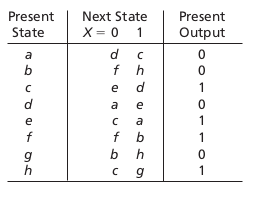## Implication tables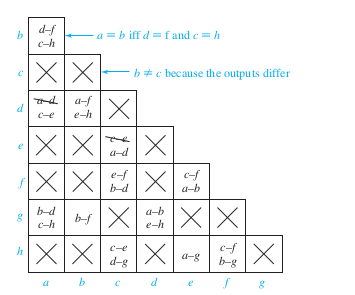## Thanks, Questions, Feedback?

https://vikasdhiman.info/ECE275-Sequential-Logic/